### Home > PC > Chapter 9 > Lesson 9.1.4 > Problem9-56

9-56.
1. Compute the following limits using either algebra or your calculator. Homework Help ✎

1.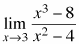2.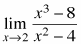3.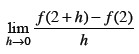when f(x) = 3x − 4.

4.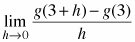when g(x) =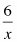.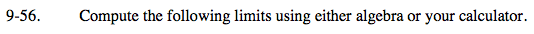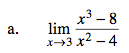Substitute 3 in for x.
You can do that because you do not have division by zero.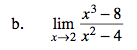Do NOT substitute 2 in for 'x' yet or you will have division by zero problem. Factor first, eliminating this problem. Then substitute 2 in for 'x'.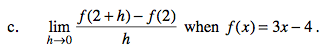$\lim_{x\rightarrow 0}\frac{(3(2+h)-4)-((3)(2)-4)}{h}$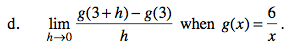$\lim_{x\rightarrow 0}\frac{\frac{6}{3+h}-\frac{6}{3}}{h}=\frac{\frac{18-6(3+h)}{3(3+h)}}{h}$